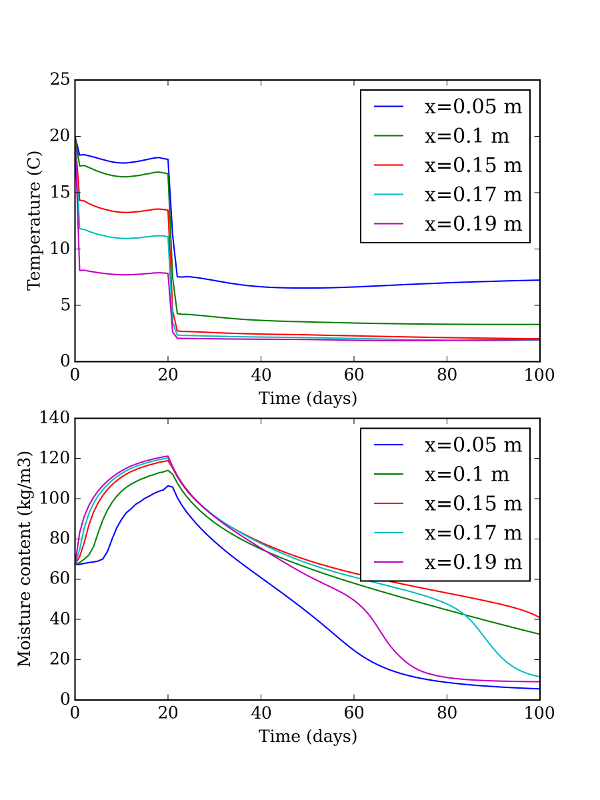This script simulates the third exercise of the Hamstad benchmark package: transient heat and moisture transfer impacted by air flow through a lightweight wall.

Note that the external air pressure fluctuates on one side of the domain, and that its values are read from a .txt file which has two columns labeled `Time (s)` and `DeltaP`. This file is available in the `hamopy/benchmarks` folder

## Script

``````import numpy  as np
import pandas as pd
import matplotlib.pylab as plt

# All things necessary to run the simulation
from hamopy import ham_library as ham
from hamopy.classes import Mesh, Boundary, Time
from hamopy.algorithm import calcul
from hamopy.postpro import evolution

# Choice of materials and geometry
from hamopy.materials.hamstad import BM3
mesh = Mesh(**{"materials"    : [BM3],
"sizes"        : [0.2],
"nbr_elements" :  })

# Boundary conditions
clim_file = 'BM3 climate.txt'
clim1 = Boundary('Fourier',**{"file"      : clim_file,
"time"      : "Time (s)",
"T"         : 293.15,
"HR"        : 0.7,
"h_t"       : 10,
"h_m"       : 2e-7,
"P_air"     : "DeltaP"})

clim2 = Boundary('Fourier',**{"T"         : 275.15,
"HR"        : 0.8,
"h_t"       : 10,
"h_m"       : 7.38e-12 })
clim = [clim1, clim2]

# Initial conditions
init = {'T'  : 293.15,
'HR' : 0.95}

# Time step control
time = Time('variable',**{"delta_t"  : 900,
"t_max"    : 8640000,
"iter_max" : 12,
"delta_min": 1e-3,
"delta_max": 900 } )

# Calculation
results = calcul(mesh, clim, init, time)

# Post processing: what time and coordinate scales we wish to display the results on
data0 = pd.read_csv(clim_file, delimiter='\t')
t_out = np.array( data0['Time (s)'] )
x_out = [0.05, 0.1, 0.15, 0.17, 0.19]

# Use the evolution function to extract the temperature and humidity profiles
Temperature = np.column_stack([evolution(results, 'T', _, t_out) for _ in x_out])
Humidity = np.column_stack([evolution(results, 'HR', _, t_out) for _ in x_out])
MoistureContent = BM3.w(ham.p_c(Humidity, Temperature), Temperature)

# Plotting results
fig, ax = plt.subplots(2, 1)
ax.plot(t_out / (24 * 3600), Temperature)
ax.set_xlabel('Time (days)')
ax.set_ylabel('Temperature (C)')
ax.legend(('x=0.05 m', 'x=0.1 m', 'x=0.15 m', 'x=0.17 m', 'x=0.19 m'))
ax.plot(t_out / (24 * 3600), MoistureContent)
ax.set_xlabel('Time (days)')
ax.set_ylabel('Moisture content (kg/m3)')
ax.legend(('x=0.05 m', 'x=0.1 m', 'x=0.15 m', 'x=0.17 m', 'x=0.19 m'))
plt.show()
``````

## Results